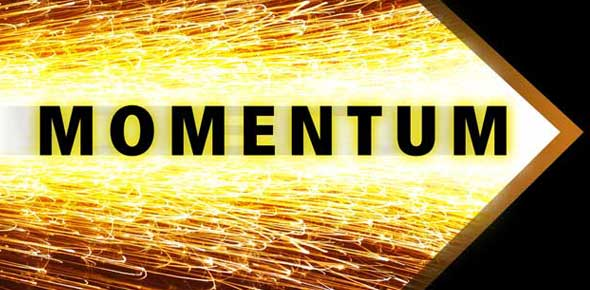# Momentum & Impulse

5 Questions | Total Attempts: 1189SettingsShort quiz on momentum and impulse.

• 1.
Momentum
• A.

Is the inertia of objects in motion

• B.

Depends on an object's velocity

• C.

Is a vector quantity

• D.

All of the above

• 2.
Which of the following probably has the largest momentum?
• A.

SUV speeding down the highway

• B.

Santa sliding slowly down the chimney

• C.

Centennial gym

• D.

Mack truck parked on the side of the road

• 3.
It is correct ot say that impulse is equal to
• A.

Momentum

• B.

The force multiplied by the distance the force acts

• C.

The change in momentum

• D.

All of these

• 4.
When a gun is fired the momentum of the the bullet
• A.

Is less than the momentum of the gun and in the same direction

• B.

Is greater than the momentum of the gun and in the opposite direction

• C.

Is equal to the momentum of the gun and in the opposite direction

• D.

Is equal to the momentum of the gun and in the same direction

• 5.
Padded dash boards in cars are safer in an accident than non padded ones because an occupant hitting the dash has (CHECK ALL THAT APPLY)
• A.

Increased time of impact

• B.

Decreased impulse

• C.

Decreased impulse force

• D.

Decreased change in momentum

• E.

Decreased acceleration

Related TopicsBack to top